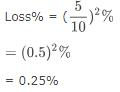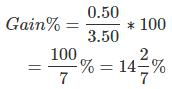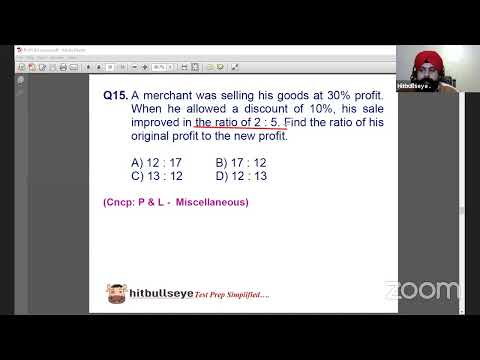Courses

# Test: Profit & Loss- 2

## 10 Questions MCQ Test UPSC CSAT Preparation | Test: Profit & Loss- 2

Description
This mock test of Test: Profit & Loss- 2 for Quant helps you for every Quant entrance exam. This contains 10 Multiple Choice Questions for Quant Test: Profit & Loss- 2 (mcq) to study with solutions a complete question bank. The solved questions answers in this Test: Profit & Loss- 2 quiz give you a good mix of easy questions and tough questions. Quant students definitely take this Test: Profit & Loss- 2 exercise for a better result in the exam. You can find other Test: Profit & Loss- 2 extra questions, long questions & short questions for Quant on EduRev as well by searching above.
QUESTION: 1

### A man sells two houses at the rate of Rs.1.995 lakhs each. On one he gains 5% and on the other, he loses 5%. What is his gain or loss percent in the whole transaction?

Solution:

The correct answer is A asQUESTION: 2

### A trader gives 12% additional discount on the discounted price, after giving an initial discount of 20% on the labeled price of an item. The final sale price of the item is Rs.704. Find out the labeled price?

Solution:

Let the marked price be Rs. 100.
Successive discounts of 20% and 12% were given.
Sale price = 100 × 80/100 × 88/100 = Rs.704/10
If the sale price is Rs.704/10, the marked price is Rs.100
If the sale price is Rs.704, the marked price is
= 100 × 10 × 704/704 =  Rs.1000

QUESTION: 3

### A trader mixes 26 kg of rice at Rs. 20 per kg with 30 kg of rice of other variety at Rs. 36 per kg and sells the mixture at Rs. 30 per kg. What is his profit percentage?

Solution:

C.P. of 56 kg rice = Rs. (26 x 20 + 30 x 36) = Rs. (520 + 1080) = Rs. 1600.

S.P. of 56 kg rice = Rs. (56 x 30) = Rs. 1680.

Gain =(80/1600*100) % = 5%

QUESTION: 4

On selling 17 balls at Rs. 720, there is a loss equal to the cost price of 5 balls. What is the cost price of a ball?

Solution:

Let the cost price of a ball be Rs. x.

So, the CP of 17 balls is Rs. 17x.

The CP of 5 balls is Rs. 5x.

Since Loss = CP - SP

So, 5x = 17x - 720

On solving, we get

12 x = 720

that is, x = 60

So, the cost price of a ball is Rs. 60.

QUESTION: 5

If a material is sold for Rs.34.80, there is a loss of 25%. Find out the cost price of the material?

Solution:

The correct answer is A as
cost price
=34.80×100/75
=46.40

QUESTION: 6

A shopkeeper sells one more radio for Rs. 840 at a gain of 20% and another for Rs. 960 at a loss of 4%. What is his total gain or loss percentage?

Solution:

Case 1) S.P of transistor = Rs 840
Profit = 20%
By unitary method C.P = (100/120) ×840= 700Rs
Case 2) S.P of transistor = Rs960
Loss = 4%
By unitary method C.P = (100/96) × 960 = 1000 Rs
Total C.P = 700 + 1000 =1700 Rs
Total S.P= 840 +960 = 1800Rs
There is a profit of = 1800 - 1700 = Rs100
Therefore profit% = (100/1700) × 100 = 100/17
= 5.882% Ans

QUESTION: 7

John purchased a machine for Rs. 80,000. After spending Rs.5000 on repair and Rs.1000 on transport he sold it with 25% profit. What price did he sell the machine?

Solution:

C.P. = (80000 + 5000 + 1000)
⇒ 86000
As we know that Profit = 25%
S.P. = 125% of 86000
⇒ (125/100 * 86000)
⇒ 107500 Rs.

QUESTION: 8

100 oranges were brought at the rate of Rs. 350 and sold at the rate of Rs. 48 per dozen. What is the percentage of profit or loss?

Solution:

So before solving this question we will get the C.P. and S.P. of 1 article to get the gain percent.

C.P. of 1 orange = 350/100 = Rs 3.50
S.P. of one orange = 48/12 = Rs 4 [note: divided by 12 as 1 dozen contains 12 items]
Gain = 4 - 3.50 = Rs 0.50Profit

QUESTION: 9

When an item is sold for Rs. 18,700, the owner loses 15%. At what price should that plot be sold to get a gain of 15%?

Solution:

85 : 18700 = 115 : x
⇒ x = (18700*115)/85
x = 25300

QUESTION: 10

A fruit seller sells apples at the rate of Rs.9 per kg and thereby loses 20%. At what price per kg, he should have sold them to make a profit of 5%?

Solution:

Track your progress, build streaks, highlight & save important lessons and more!

### Similar Content### Related tests